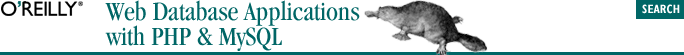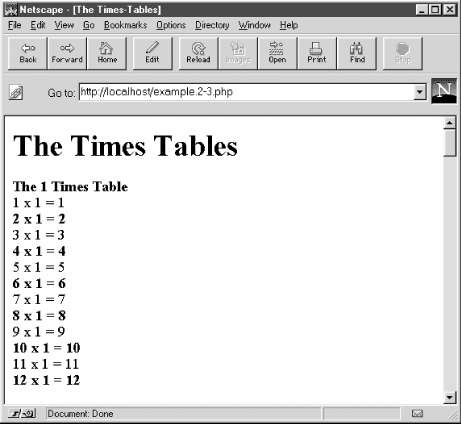home | O'Reilly's CD bookshelfs | FreeBSD | Linux | Cisco | Cisco Exam## 2.4. A Working Example

In this section, we use the techniques described so far to develop a simple, complete PHP script. The script doesn't process input from the user, so we leave some of the best features of PHP as a web scripting language for discussion in later chapters.#### Figure 2-2. The output of the times-tables script shown rendered in a Netscape browser

```<html>
<title>The Times-Tables</title>
<body bgcolor="#ffffff">
<h1>The Times Tables</h1>
<p><b>The 1 Times Table</b>
<br>1 x 1 = 1
<br><b>2 x 1 = 2</b>
<br>3 x 1 = 3
<br><b>4 x 1 = 4</b>
<br>5 x 1 = 5```

The script produces this output using a mixture of HTML and an embedded PHP script.

The completed PHP script and HTML to produce the times tables are shown in Example 2-3. The first nine lines are HTML that produces the <head> components and the <h1>The Times Tables</h1> heading at the top of the web page. Similarly, the last two lines are HTML that finishes the document: </body> and </html>.

Between the two HTML fragments that start and end the document is a PHP script to produce the times-table content and its associated HTML. The script begins with the PHP open tag <?php and finishes with the close tag ?>.

#### Example 2-3. A script to produce the times tables

```<!DOCTYPE HTML PUBLIC
"-//W3C//DTD HTML 4.0 Transitional//EN"
"http://www.w3.org/TR/html4/loose.dtd" >
<html>
<title>The Times-Tables</title>
<body bgcolor="#ffffff">
<h1>The Times Tables</h1>
<?php
// Go through each table
for(\$table=1; \$table<13; \$table++)
{
echo "<p><b>The " . \$table . " Times Table</b>\n";

// Produce 12 lines for each table
for(\$counter=1; \$counter<13; \$counter++)
{

// Is this an even-number counter?
if (\$counter % 2 == 0)
// Yes, so print this line in bold
echo "<br><b>\$counter x \$table = " .

else
// No, so print this in normal face
echo "<br>\$counter x \$table = \$answer";
}
}
?>
</body>
</html>```

The script is designed to process each times table and, for each table, to produce a heading and 12 lines. To do this, the script consists of two nested loops: an outer and inner for loop.

The outer for loop uses the integer variable \$table, which is incremented by 1 each time the loop body is executed until \$table is greater than 12. The body of the outer loop prints the heading and executes the inner loop that actually produces the body of each times table.

The inner loop uses the integer variable \$counter to generate the lines of the times tables. Inside the loop body, the \$answer to the current line is calculated by multiplying the current value of \$table by the current value of \$counter.

Every second line of the tables and the times-table headings are encapsulated in the bold tag <b> and bold end tag </b>, which produces alternating bold lines in the resulting HTML output. After calculating the \$answer, an if statement follows that decides whether the line should be output in bold tags. The expression the if statement tests uses the modulo operator % to test if \$counter is an odd or even number.

The modulo operation divides the variable \$counter by 2 and returns the remainder. So, for example, if \$counter is 6, the returned value is 0, because 6 divided by 2 is exactly 3 with no remainder. If \$counter is 11, the returned value is 1, because 11 divided by 2 is 5 with a remainder of 1. If \$counter is even, the conditional expression:

` (\$counter % 2 == 0) `

is true, and bold tags are printed.

### 2.4.1. Comments on Example 2.3

Example 2-3 is complete but isn't especially interesting. Regardless of how many times the script is executed, the result is the same web page. In practice, you might consider running the script once, capturing the output, and saving it to a static HTML file. If you save the output as HTML, the user can retrieve the same page, with less web-server load and a faster response time.

In Chapter 4, we introduce more PHP scripts that don't support input from the user. However, the difference is that the scripts interact with the MySQL DBMS and run SQL queries. The result is that the pages can change if the underlying data in the database is updated. Therefore, unlike our simple example here, the scripts in Chapter 4 may not be readily replaced with static HTML pages.